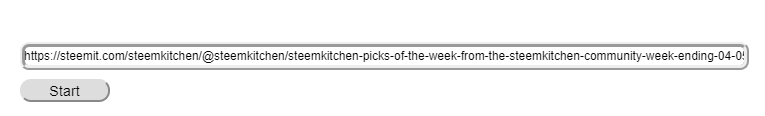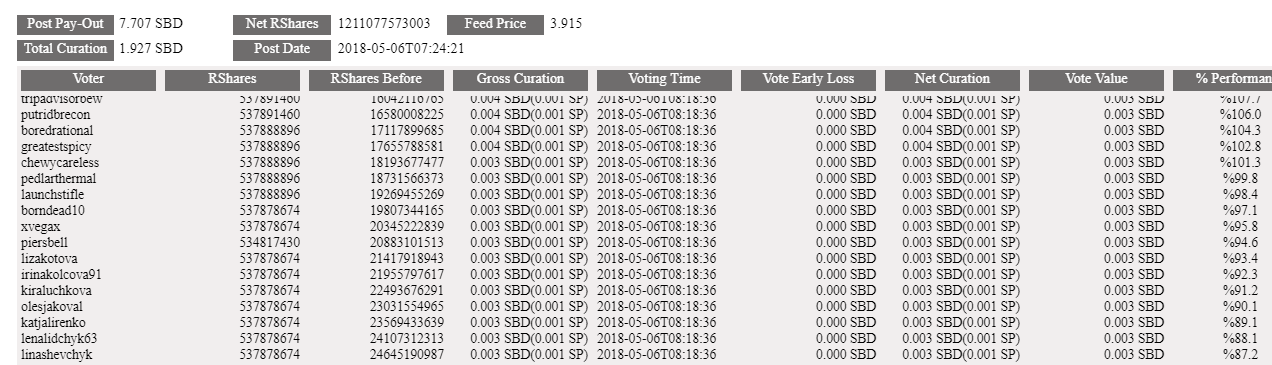# Curation Break-Down tool

in #utopian-io4 years ago

#### Repository

https://github.com/firedreamgames/curation_break_down

Curation Break Down is a tool made to analyze the actual or pending curation rewards in a post.

The tool gives :• Actualized or pending post pay-out
• Actualized or pending total curation on the post
• The net total rshares on the post
• Post date
• Feed-price ( used for converting SBD to Steem )
• All the voters on the post
• Curation reward for each user and their votes cast
• Curation performance ( curation received/vote value )

This is an important tool to see the impact of curation trails on certain posts and to estimate the actions on curation maximization.

### How to use Curation Break Down tool• Press “Start” button• The tool will show all the post and voters data on the screen.### Code

The full code can be found in GitHub
The explanations for each line of code is also implemented

``````steem.api.setOptions({
url: 'https://api.steemit.com'
});

var p_date;
var p_date_parsed;
var post_pay_sbd;
var curation_pay_sbd;
var total_pay_sbd;
var is_paid;
var feed;
var total_net_rshares;
document.body.style.zoom = "90%";

// get feed history from STEEM.API  This will be used to get current feed price ( SBD-to-SP coversion )
steem.api.getFeedHistory(function(err, res) {
feed = parseFloat(res.current_median_history.base);

});

// function for clear all the fields
var clearSearchFields = function() {
\$('#voter,#rshares,#rshares_before,#curation_wo_penalty,#vote_time,#SP_early_penalty,#SP_curation,#vote_sp,#performance,#post_pay,#feed_price,#post_date,#curation,#net_shares').html('');
}

// Starting function
function Start_Analysis() {
clearSearchFields();
var perm = link.split("/"); //split the url to get permlink and user
var length_perm = perm.length;
var permlink = perm[length_perm - 1]; //the permlink is the last element after "/" sign
var user_raw = perm[length_perm - 2]; //form the array to find the user to be voted - user is found at the one before final element of array
var usmat = user_raw.split("@"); //we need the username without @sign, so split the raw array
var author = usmat[usmat.length - 1]; //user name is the last element of the array

steem.api.getContent(author, permlink, function(err, result) { // get values from steem.api for the post content
p_date = result.created;
p_date_parsed = Date.parse(p_date);// get posting time as time-stamp

if (parseFloat(result.vote_rshares) == 0) {// check if the post is already paid. The curation calculation differs
is_paid = true;//this is to be used later for improvements

// If the post is already paid, all curation and post payments can be taken from block-chain
post_pay_sbd = parseFloat(result.total_payout_value).toFixed(3);
curation_pay_sbd = parseFloat(result.curator_payout_value).toFixed(3);
total_pay_sbd = parseFloat(post_pay_sbd) + parseFloat(curation_pay_sbd);
}

if (parseFloat(result.vote_rshares) != 0) {
is_paid = false;//this is to be used later for improvements
// If the post is fresh - not paid, we use estimation method for calculating curation rewards.
post_pay_sbd = (parseFloat(result.pending_payout_value) * 0.75).toFixed(3);// post pay-out is %75 of total pay-out
curation_pay_sbd = (parseFloat(result.pending_payout_value) * 0.25).toFixed(3);// curation pay-out is %25 of total pay-out
total_pay_sbd = parseFloat(post_pay_sbd) + parseFloat(curation_pay_sbd); // total pay-out
}
calculate();// send the result to calculation
});
}

// function to perform all the calculation
function calculate() {
total_net_rshares = 0;
var before = 0;
var now;
var voter = [];
var voter_before = [];
var voter_now = [];
var v_time = [];
var time_diff = [];
var gross_curation = [];
var penalty = [];
var net_curation = [];
var vote_value = [];
var r_shares = [];

votes.sort(compare);// this is important, votes must be sorted according to their voting time
for (let i = 0; i < votes.length; i++) {
total_net_rshares = total_net_rshares + parseInt(votes[i].rshares);//calculate total net rshares.( If the post is paid, this is needed )
r_shares.push(votes[i].rshares);// get rshares of all the voters
}

//console.log(total_net_rshares);
var sbd_per_shares = total_pay_sbd / total_net_rshares;// calculate SBD value per share - to calculate vote value of each voter

before = 0;
for (let i = 0; i < votes.length; i++) {
voter_before.push(before);
voter_now.push(now);
var ratio = (Math.sqrt(now) - Math.sqrt(before)) / (Math.sqrt(total_net_rshares));// main sqrt calculation for curation reward - find the ratio per voter
var gc=curation_pay_sbd * ratio
if(gc<0){// if it is a downvote, curation earned will be not negative but zero
gc=0;
}

gross_curation.push(gc);// gross curation - as voting time penalty unapplied - put in the array.

var v_date_parsed = Date.parse(v_date);// get voting time as time-stamp

var vote_late = (v_date_parsed - p_date_parsed) / (30 * 60 * 1000);// check the difference with 30 minutes.
if (vote_late >= 1) {// if it is over 30 minutes no penalty applied.
vote_late = 1;
}
var curation_loss = (gc * (1 - vote_late));// according to difference found, apply the penalty and calculate curation loss
penalty.push(curation_loss);
net_curation.push(gc - curation_loss);// net curation is gross curation (gc) - curation_loss
before = now;// set the total rshares before for next calculation
}
// send all the results to to_div function for showing.
to_div(voter, r_shares, voter_before, voter_now, v_time, vote_value, gross_curation, penalty, net_curation);
}

//function to show results.
function to_div(voter, r_shares, voter_before, voter_now, v_time, vote_value, gross_curation, penalty, net_curation) {
document.getElementById("post_pay").innerHTML = parseFloat(total_pay_sbd).toFixed(3) + " SBD";
document.getElementById("curation").innerHTML = parseFloat(curation_pay_sbd).toFixed(3) + " SBD";
document.getElementById("net_shares").innerHTML = total_net_rshares;
document.getElementById("feed_price").innerHTML = feed;
document.getElementById("post_date").innerHTML = p_date;
for (let i = 0; i < voter.length; i++) {
document.getElementById("voter").innerHTML = document.getElementById("voter").innerHTML + voter[i] + "<br />";
document.getElementById("rshares").innerHTML = document.getElementById("rshares").innerHTML + r_shares[i] + "<br />";
document.getElementById("rshares_before").innerHTML = document.getElementById("rshares_before").innerHTML + voter_before[i] + "<br />";
document.getElementById("curation_wo_penalty").innerHTML = document.getElementById("curation_wo_penalty").innerHTML + gross_curation[i].toFixed(3) + " SBD" + "(" + (gross_curation[i] / feed).toFixed(3) + " SP)" + "<br />";
document.getElementById("vote_time").innerHTML = document.getElementById("vote_time").innerHTML + v_time[i] + "<br />";
document.getElementById("SP_early_penalty").innerHTML = document.getElementById("SP_early_penalty").innerHTML + penalty[i].toFixed(3) + " SBD" + "<br />";
document.getElementById("SP_curation").innerHTML = document.getElementById("SP_curation").innerHTML + net_curation[i].toFixed(3) + " SBD" + "(" + (net_curation[i] / feed).toFixed(3) + " SP)" + "<br />";
document.getElementById("vote_sp").innerHTML = document.getElementById("vote_sp").innerHTML + vote_value[i].toFixed(3) + " SBD" + "<br />";
document.getElementById("performance").innerHTML = document.getElementById("performance").innerHTML + "%" + ((net_curation[i] / vote_value[i]) * 100).toFixed(1) + "<br />";

}

}

function compare(a, b) {
const timeA = a.time;
const timeB = b.time;
let comparison = 0;
if (timeA > timeB) {
comparison = 1;
} else if (timeA < timeB) {
comparison = -1;
}
return comparison;
}
``````

This tool shows that, there is a way to get curation rewards equal to or higher than self-upvoting with micro votes.
The aim of this tool is to analyze the voting behavior, especially on the trending posts.

Using this tool, I will further study the curation and voting behavior and get an explanation to micro votes we see on most of the posts.

### Connect

@FireDream - Steemit

@firedream#3528 - Discord

Curation break-down tool : https://fdsteemtools.neocities.org/curation_break_down.html
GitHub: https://github.com/firedreamgames/curation_break_down

### Proof of WorkSort:

Thank you for your contribution, it has been approved. You can create one application and all these features in that instead of creating one by one.

Need help? Write a ticket on https://support.utopian.io.
Chat with us on Discord.

[utopian-moderator]

@codingdefined,
FD.

Thank you for the tool, @firedream: I find it useful!

I would like to automate fulfilling of the link section with the URL address of the page I'm currently browsing clicking on a Curation_BreakDown bookmark created on my bookmark toolbar: could you provide a javascript to do that?

@inlakech, thank you for finding it useful...I will try to learn and apply what you recommend.
FD.

Thank you, very kind! :)

Hey @firedream
Thanks for contributing on Utopian.

Contributing on Utopian
Learn how to contribute on our website or by watching this tutorial on Youtube.